# DAV Class 5 Maths Chapter 7 Worksheet 8 Solutions

The DAV Maths Book Class 5 Solutions and DAV Class 5 Maths Chapter 7 Worksheet 8 Solutions of Multiplication and Division of Decimal Numbers offer comprehensive answers to textbook questions.

## DAV Class 5 Maths Ch 7 Worksheet 8 Solutions

Question 1.
Find the quotient orally.
(a) 1.7 ÷ 10
Solution:
1.7 ÷ 10 = 0.17
Decimal point shift one place to left.

(b) 4.9 ÷ 10
Solution:
4.9 ÷ 10 = 0.49
Decimal point shift one place to left.

(c) 19.2 ÷ 1000
Solution:
19.2 ÷ 1000 = .0192
The decimal point shifts three places to the left.(d) 57.98 ÷ 100
Solution:
57.98 ÷ 100 = 0.5798
The decimal point shifts two places to the left.

(e) 601.8 ÷ 1000
Solution:
601.8 ÷ 1000 = 0.6018
The decimal point shifts three places to the left.

(f) 44.81 ÷ 1000
Solution:
44.81 ÷ 1000 = 0.04481
The decimal point shifts three places to the left.

(g) 1.3 ÷ 100
Solution:
1.3 ÷ 100 = 0.013
The decimal point shifts two places to the left.

(h) 2.56 ÷ 1000
Solution:
2.56 ÷ 1000 = 0.00256
The decimal point shifts three places to the left.(i) 148.5 ÷ 10
Solution:
148.5 ÷ 10 = 14.85
Decimal point shift one place to left.

(j) 708.13 ÷ 100
Solution:
708.13 ÷ 100 = 7.0813
The decimal point shifts two places to the left.

Question 2.
Fill in the boxes. The first one is done for you.
(a)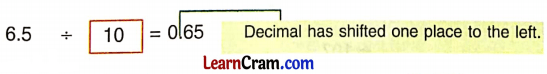Solution:
6.5 ÷ 10 = 0.65
Decimal has shifted 1 one place to the left.

(b)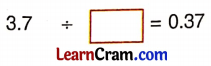Solution:
3.7 ÷ 10 = 0.37
(Decimal has shifted one place to the left)(c)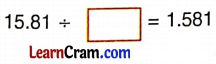Solution:
15.81 ÷ 10 = 1.581
(Decimal has shifted one place to the left)

(d)Solution:
8.19 ÷ 10 = 0.819
(Decimal has shifted one place to the left)

(e)Solution:
77.1 ÷ 100 = 0.771
(Decimal has shifted two places to the left)

(f)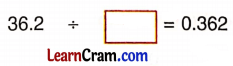Solution:
36.2 ÷ 100 = 0.362
(Decimal has shifted two places to the left)(g)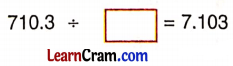Solution:
710.3 ÷ 100 = 7.103
(Decimal has shifted two places to the left)

DAV Class 5 Maths Chapter 7 Worksheet 8 Notes

In order to divide a decimal number by 10, 100, 1000 we just shift the decimal point in the quotient to the left by as many places as there are zeroes in the divisor.

Examples:
(a) 76.9 ÷ 10 = 7.69
The decimal point shifts one place to the left.

(b) 349.6 ÷ 100 = 3.496
The decimal point shifts two places to the left.(c) 756.3 ÷ 1000 = 0.7563
The decimal point shifts three places to the left.